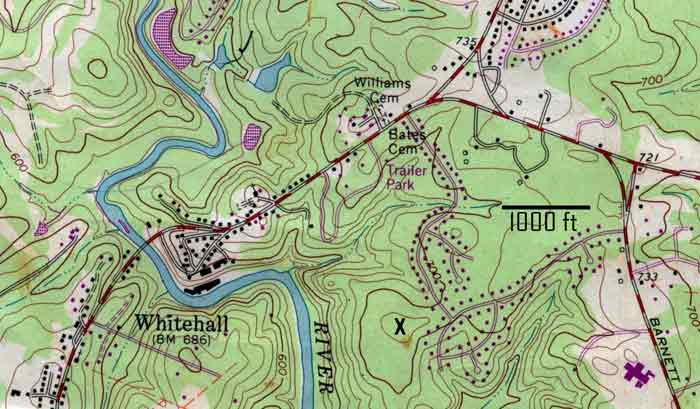# Maps, Scales & Slope

 Remember that a map scale is a ratio of distance on map to distance on ground, using same units. So 1:24,000 means 1” (inch) on the map equals 24,000” on the ground; then just convert 24,000” to feet: 24,0000” x 1ft/12” = 2,000 ft or, 1” = 2,000′. A. On a map of scale 1:36,000, two points are separated by 4”. How many feet is this on the ground? B. Athens is 12 miles from my house. How many inches would this be on a 1:50,000 scale map? Areas, of course, can be calculated on maps by converting map distances to feet (or miles), then multiplying. A one inch by one inch square on a 1:24,000 map would be 2,000 x 2,000 = 4,000,000 square feet. You can convert to acres, or square miles, using the appropriate conversion. C. A field on a 1:24,000 map is about 2” wide by 4” long. Calculate the area in acres. D. On a 1:15,000 map, how big of a square (in inches) would a 1-acre home lot be? E. The Oconee River watershed is approximately 6” by 20” on a 1:500,000 scale map. What is its area in square miles? Slope in most landscape work is expressed as rise over run, given as a percentage (“% slope”). A 5% slope has a rise (difference in elevation) of 5′ per every 100′ of horizontal distance (“run”). You get “runs” by using the map scale; rises come from topo maps, where you read elevations or you can count contour lines to get the elevation difference. F. Two points are 2,200 feet apart; one has an elevation of 760′, the other 540′. Calculate the average slope between these points. G. On a 1:30,000 map two points are separated by 6”; there are 6 contour lines between the two points (the map contour interval is 20′). Calculate the slope. Use the topo map below to answer questions H, I & J. The contour interval is 20 ft., and a 1000 ft. scale is shown on the map.H. How many ft is it from the spot marked “x” and the purple building to the east located on Barnett Rd.? I. Calculate the % slope between the “x” on the top of the hill and the Oconee River directly to the west. J. Draw an imaginary rectangle around the town of Whitehall which encloses most of the houses there. What is the area of the town?